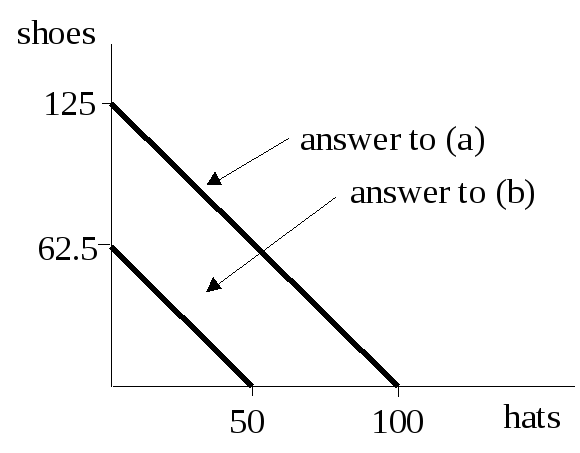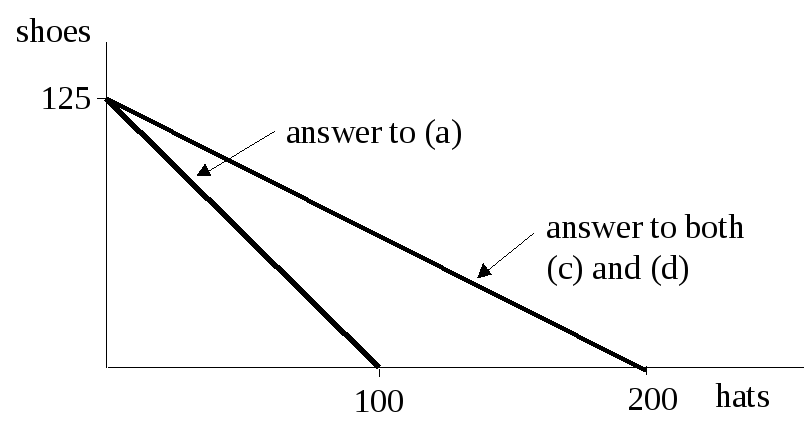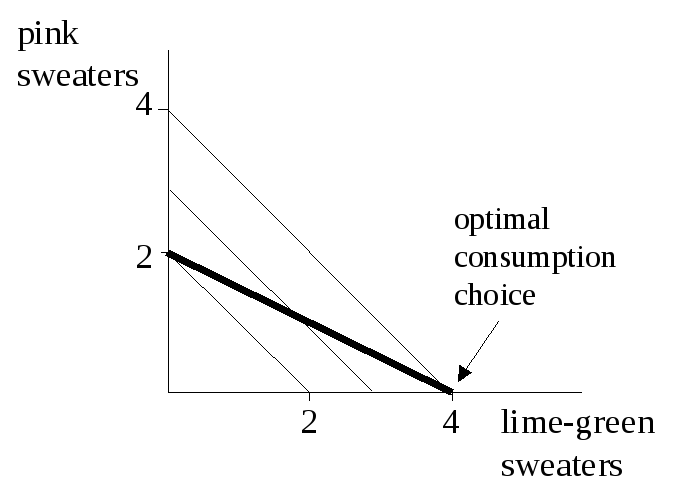# Lecture 3 Practice Question Answers

 Lecture 3 Practice Question Answers From Browning and Zupan: See graphs below. I have included the answer to (a) in both graphs so you can compare the results. Notice that parts (c) and (d) have the same answer.3.3. Answer in book. Note: the answer for the slope assumes that hamburgers are on the vertical axis and french fries are on the horizontal axis. 3.9. Answer in book. However, notice that the question says MRS = 1C/1F. That’s an okay way to say it if champagne is on the vertical axis. But if champagne is on the horizontal axis, as in the book’s answer, then you should say MRS = 1F/1C. (For this particular MRS it doesn’t matter much, because MRS = 1 either way no matter which good is on the vertical axis. But it matters when MRS does not equal 1. At the optimal point, MRS = 3 if champagne is on the horizontal axis as in the book’s answer, but MRS = 1/3 if champagne is on the vertical axis.) 3.17. (a) See graph of Thurston’s budget constraint and indifference curves on the next page. The darker line is the budget constraint, and the lighter lines are indifference curves. The indifference curves are straight lines because the goods are perfect substitutes (Thurston doesn’t care what color sweater he wears). Notice that the optimal consumption choice is to buy only lime-green sweaters.Share with your friends: